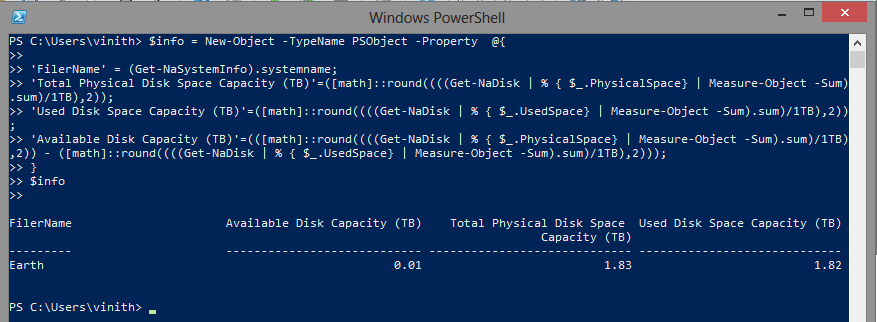Microsoft Virtualization Discussions

Highlighted

## Get total filer capacity and available capacity

I am new to powershell and am trying to create a script that will give the total capacity of the filer and available capacity.

I have seen the getnavol and getaggr and use them to get that data but am stumped on how to get total capacity data.

Any assistance is appreciated

Highlighted

## Re: Get total filer capacity and available capacityHi Craig, We can attain the same using powershell custom objects.

Have a look at the code below and run it in your environment to get the required information. We can use Get-NaDisk and extract the total,available and used storage on the controller

\$info = New-Object -TypeName PSObject -Property  @{

'FilerName' = (Get-NaSystemInfo).systemname;

'Total Physical Disk Space Capacity (TB)'=([math]::round((((Get-NaDisk | % { \$_.PhysicalSpace} | Measure-Object -Sum).sum)/1TB),2));

'Used Disk Space Capacity (TB)'=([math]::round((((Get-NaDisk | % { \$_.UsedSpace} | Measure-Object -Sum).sum)/1TB),2));

'Available Disk Capacity (TB)'=(([math]::round((((Get-NaDisk | % { \$_.PhysicalSpace} | Measure-Object -Sum).sum)/1TB),2)) - ([math]::round((((Get-NaDisk | % { \$_.UsedSpace} | Measure-Object -Sum).sum)/1TB),2)));

}

\$infoWe use custom hash tables to append all values to one place rather than using Add-Content to create reusable objects, please have a look at the link http://blogs.msdn.com/b/powershell/archive/2009/12/05/new-object-psobject-property-hashtable.aspx

Please do let me know if this is what you need.

Forums